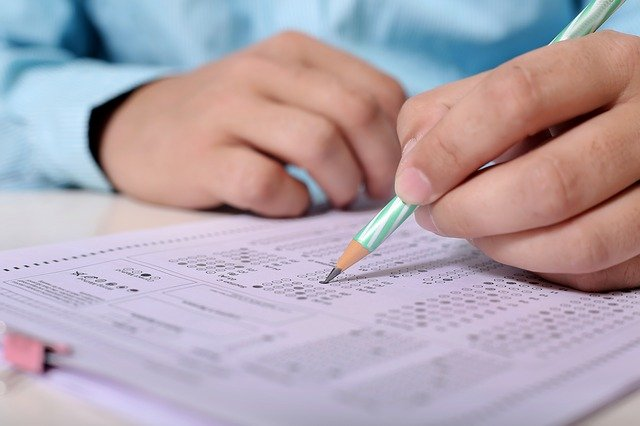# Format of O Level Additional Mathematics (syllabus 4049)The Singapore O Level Additional Mathematics (also called A- Math or Add- Math) consists of 2 papers. Any of the topics in the Add Math syllabus can come out in paper 1 or 2. But generally, if a particular concept is already tested in paper 1, then it will not be tested in paper 2. Also, all questions are compulsory, so don’t skip any topics when revising!

### The Two Papers in O Level Additional Math (Syllabus 4049)

A summary of the weightage, duration and marks for paper 1 and paper 2 of Add Math are as follows:

Notice that both papers have equal weightage and are essentially similar in the number of marks and duration?
The only difference between the 2 papers are the number of marks per question and the number of questions in the paper. In paper 1, there will be 12 – 14 questions of varying marks and lengths, up to 10 marks per question. In paper 2, there will be 9 – 11 questions of varying marks and lengths, up to 12 marks per question. All questions in both papers are compulsory.

### How to prepare for Paper 1 and Paper 2 (O Level Additional Mathematics Exam)

You’ll have the exam for paper 1 first before paper 2.

You would have noticed that there is not much difference between paper 1 and paper 2. Hence, you should revise the entire O Level Additional Mathematics syllabus when preparing for paper 1. You should also try both papers 1 and 2 of past exam questions from your ten-year series and prelim papers when preparing for paper 1 as what was tested in paper 2 previously could come up in paper 1.

Paper 2 comes after paper 1. Studying for paper 2 should be simply revision since you have already studied for paper 1. For paper 2, focus on the concepts that have yet been tested, since they are unlikely to ask the same type of question twice.

### Mock Papers for Syllabus 4049

2021 is the first year the Additional Mathematics Syllabus (syllabus 4049) is tested. SEAB has provided specimen papers for both paper 1 and paper 2 on their website, which you can download. I have provided the link below:

Do download and try them before the exams, so that you are familiar with the format of the exam.

## Learn O Level A Math on-demand

Want to learn O Level A Math on-demand? Check out our course here.

error: Content is protected !!